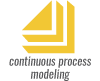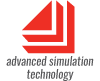# Modeling Methodologies

The formalism used to specify a system is termed a modeling methodology. Continuous modeling (sometimes known as process modeling) is used to describe a flow of values. Discrete Event models track unique entities. Discrete Rate models share some aspects of both continuous and discrete event modeling.

In all three types of simulations, what is of concern is the granularity of what is being modeled and what causes the state of the model to change.

## Continuous

The time step is fixed at the beginning of the simulation, time advances in equal increments, and values change based directly on changes in time. In this type of model, values reflect the state of the modeled system at any particular time, and simulated time advances evenly from one time step to the next.

For example, an airplane flying on autopilot represents a continuous system since its state (such as position or velocity) changes continuously with respect to time. Continuous simulations are analogous to a constant stream of fluid passing through a pipe. The volume may increase or decrease at each time step, but the flow is continuous.

## Discrete Event

The system changes state as events occur and only when those events occur; the mere passing of time has no direct effect on the model. Unlike a continuous model, simulated time advances from one event to the next and it is unlikely that the time between events will be equal.

A factory that assembles parts is a good example of a discrete event system. The individual entities (parts) are assembled based on events (receipt or anticipation of orders). Using the pipe analogy for discrete event simulations, the pipe could be empty or have any number of separate buckets of water traveling through it. Rather than a continuous flow, buckets of water would come out of the pipe at random intervals.

## Discrete Rate

Discrete rate simulations are a hybrid type, combining aspects of continuous and discrete event modeling. Like continuous models they simulate the flow of stuff rather than items; like discrete event models they recalculate rates and values whenever events occur.

Using the pipe analogy for a discrete rate simulation, there is a constant stream of fluid passing through the pipe. But the rates of flow and the routing can change when an event occurs.

These 3 main modeling types are compared and contrasted in the tables below.

In some branches of engineering, the term discrete is used to describe a system with periodic or constant time steps. Discrete, when it refers to time steps, indicates a continuous model; it does not have the same meaning as discrete event or discrete rate. Continuous models in ExtendSim are stepped using constant time intervals; discrete event and discrete rate models are not.## Other Modeling Approaches

Although there are several other approaches to modeling, they usually fit within one of the three major categories. For example, System Dynamics and Bond graphs are subsets of continuous modeling, and queuing theory models are subsets of discrete event modeling.

Because of their specialized use, three specific modeling approaches - and Agent Based - are described below.

## Monte Carlo

Widely used to solve certain problems in statistics, Monte Carlo simulations provide a range of results rather than a single value. This approach can be applied to any ExtendSim model and used wherever uncertainty is a factor.

Monte Carlo modeling uses random numbers to vary input parameters for a series of calculations. These calculations are performed many times and the results from each individual calculation are recorded as an observation. The individual observations are statistically summarized, giving an indication of the likely result and the range of possible results. This not only tells what could happen in a given situation, but how likely it is that it will happen.

You build a Monte Carlo simulation in ExtendSim by incorporating random elements in a model and obtaining multiple observations. There are two ways to do this:The classical Monte Carlo method is to take a single mathematical equation or set of equations, then cause the equation to be calculated many times. In this type of simulation, time is not a factor. The entire model is run to completion and evaluated at each step; each subsequent step performs a new calculation.An alternative Monte Carlo approach, typically applied in a discrete event model, is to either divide a single simulation run into multiple sections (batch means) or run the simulation many times (multi-run analysis). Monte Carlo is incorporated by adding randomness to the model, running it many times, and analyzing the results. This method can be applied to any continuous, discrete event, or discrete rate model.

## Agent-Based

With agent-based modeling you usually do not know model dynamics in advance; instead, you obtain that information from the interaction of the agents in the model.

Agent-based models share the following characteristics:The identification of individual entities within the model.A set of rules that govern individual behavior.The premise that local entities affect each other's behavior.

 Agent-based modeling is concerned with individual entities (called "agents") that interact with other agents within their specified locality. All the agents have a set of rules to follow but they also have a degree of autonomy such that model dynamics cannot be predefined. This is because agents can have intelligence, memory, social interaction, contextual and spatial awareness, and the ability to learn. Case Study Usage Intensity in Life Cycle of Real Estate - Quantification of Usage Intensity by Simulationby Shujing Deng • Technischen Universität MünchenExtendSim agent-based model of a shopping mall used to analyze shoppers' impact on building components.

## State/Action

With state/action modeling a system is modeled as a collection of discrete states. Sometimes known as a state chart, a state/action model represents a system that responds to an event by transitioning to another state. The model is composed of a series of states where each state depends on a previous state. A state has an associated action and an event that will cause that state to change to another. The transition from one state to the next is not sequential; each state can lead to any other state.

There are rules that govern the communication and transition between the states:All states accept events.One or more states may create an event as a result of a transition by another state or group of states.A group of states can be set to transition conditionally, for instance to only change if another state or group of states achieve a specific stage. These are known as guard conditions.

State/action models are independent of any of the three modeling methodologies (continuous, discrete event, or discrete rate.) They are useful for specification and verification in many areas, from computer programs to business processes.

## Tables

### Comparison Table

 Modeling Method Continuous Discrete event Discrete rate ExtendSim library Value library Electronics library Item library Rate library What is modeled Processes Individual items Flows of stuff Examples Processes: chemical, biological, economic, electronic, geological. Things: traffic, equipment, work product, people. Information: data, messages, network protocols at the packet level. Rate-based flows of stuff: homogeneous products (powders, fluids, oil, and gas), high-speed or high-volume production and packaging, data feeds and streams, mining.

### Table of continuous, discrete event, and discrete rate differences

Use this table as a guide to help determine which style to use when modeling a system.

class="tablebody" style="text-align: center;">

 Factor Continuous Discrete event Discrete rate What is modeled Values that flow through the model. Distinct entities ("items" or "things"). Bulk flows of homogeneous stuff. Or flows of otherwise distinct entities where sorting or separating is not necessary. What causes a change in state A time change An event An event Time steps Interval between time steps is constant. Model recalculations are sequential and time dependent. Interval between events is dependent on when events occur. Model only recalculates when events occur. Interval between events is dependent on when events occur. Model only recalculates when events occur. Characteristics of what is modeled Track characteristics in a database or assume the flow is homogeneous. Using attributes, items are assigned unique characteristics and can then be tracked throughout the model. Track characteristics in a database or assume the flow is homogeneous. Ordering FIFO Items can move in FIFO, LIFO, Priority, time-delayed, or customized order. FIFO Routing Values need to be explicitly routed by being turned off at one branch and turned on at the other (values can go to multiple places at the same time). By default, items are automatically routed to the first available branch (items can only be in one place at a time). Flow is routed based on constraint rates and rules that are defined in the model (flow can be divided into multiple branches). Statistical detail General statistics about the system: amount, efficiency, etc. In addition to general statistics, each item can be individually tracked: count, utilization, cycle time. In addition to general statistics, effective rates, cumulative amount. Typical uses Scientific (biology, chemistry, physics), engineering (electronics, control systems), finance and economics, System Dynamics. Manufacturing, service industries, business operations, networks, systems engineering. Manufacturing of powders, fluids, and high speed, high volume processes. Chemical processes, ATM transactions. Supply chains. Recommended packageExtendSim CPExtendSim DEExtendSim Pro
ExtendSim Store